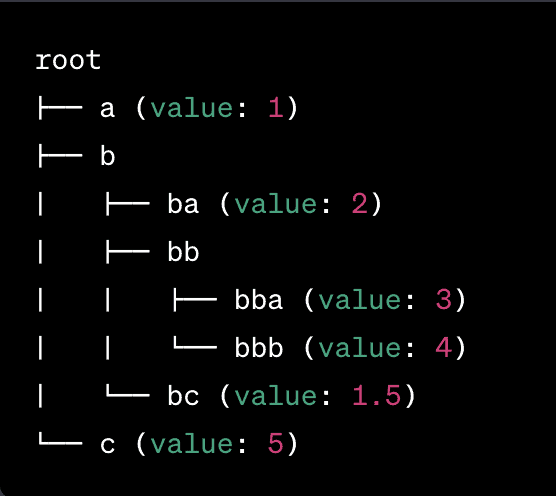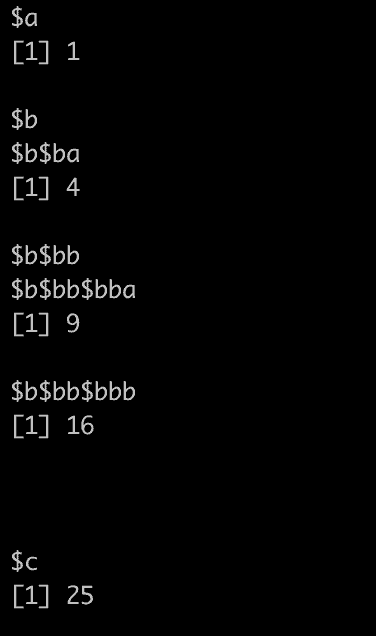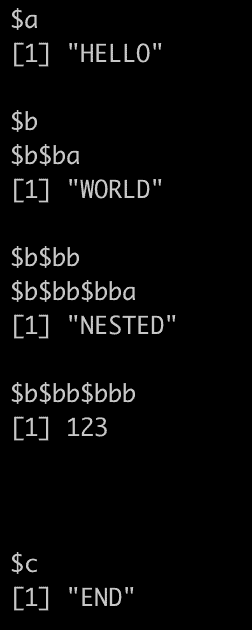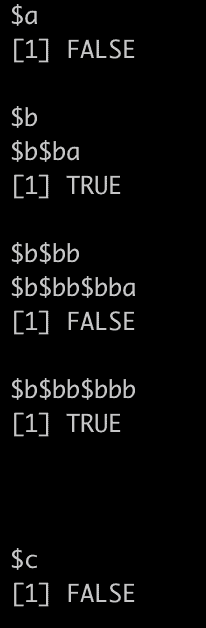# How to Use the rapply() Function in R

The rapply() function is used to apply a function to all elements of a list recursively. The rapply() is a recursive version of the lapply() function.

The rapply() function is specifically helpful when applying a function to elements of a nested list structure.

## Syntax

``````rapply(object, f, classes = "ANY", deflt = NULL,
how = c("unlist", "replace", "list"), ...)
``````

## Parameters

1. object: It represents a list or an expression.
2. f: It represents a function to be applied recursively.
3. classes: It represents the class name of the vector or “ANY” to match any of the classes.
4. deflt: It represents the default result when it is not “replace”.
5. how: It represents modes.

## Visual Representation

Imagine a tree structure where each node represents an element in the list, and branches represent the nested structure of the list.When you use rapply(), you traverse this tree, visiting each node.

Different operations depend on the “how” argument and the function you provide to rapply().

So, in essence, the rapply() function works by navigating through this tree structure and applying the given function to the nodes based on the conditions you set (like specific classes of nodes).

## Example 1: Using “replace” option

``````nested_list <- list(
a = 1,
b = list(
ba = 2,
bb = list(
bba = 3,
bbb = 4
)
),
c = 5
)
squared_list <- rapply(nested_list, function(x) x^2,
classes = "numeric", how = "replace"
)

print(squared_list)``````

Output## Example 2: Convert all character elements to uppercase in a nested list

``````# Define a nested list with character and numeric values
mixed_list <- list(
a = "hello",
b = list(
ba = "world",
bb = list(
bba = "nested",
bbb = 123
)
),
c = "end"
)

# Convert all character elements to uppercase
uppercased_list <- rapply(mixed_list, function(x) toupper(x),
classes = "character", how = "replace"
)

print(uppercased_list)
``````

Output## Example 3: Using ‘how = “list”‘: Apply a function and maintain the list structure

We need to identify which elements in our nested list are even numbers. We can use the rapply() function to return a nested list structure indicating where even numbers are.

``````# Define a nested list
nested_list <- list(
a = 1,
b = list(
ba = 2,
bb = list(
bba = 3,
bbb = 4
)
),
c = 5
)

# Identify even numbers
is_even_list <- rapply(nested_list, function(x) x %% 2 == 0,
classes = "numeric", how = "list"
)
print(is_even_list)
``````

Output## Example 4: Using unlist mode

Output

``````b.bb.bba b.bb.bbb c
3     4      5``````

That’s it!

## Related posts

sapply() function in R

apply() function in R